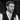# Learn the JavaScript Array.every() and Array.some() methods

Nick Scialli March 28, 2020🚀 3 minute read

If you're enjoying this blog, please consider:

`Array.every()` and `Array.some()` are handy JavaScript array methods that can help you test an array against specified criteria. In this post, we’ll quickly learn how to use them.

# Array.every()

`Array.every` takes a callback function as an argument. If the function returns `true` for each item in the array, `Array.every` returns true. Let’s check it out.

``````function test(el) {
return el < 10;
}

[1, 2, 3, 4, 5, 6].every(test);
// true``````

Since every item in the array is less than 10, the `Array.every` method returns `true`.

It’s common to pass an anonymous arrow function directly to the `every` method, so let’s do that to see a more familiar syntax:

``````[1, 2, 3, 4, 5, 6].every(el => el < 10);
// true``````

Let’s see another example. This time it’ll fail because not every element will pass the test.

``````[1, 2, 3, 4, 5, 6].every(el => el < 5);
// false``````

One nice thing that happens here is that, not only does it fail, but it exits early as soon as an element fails the test. That means it won’t even run the test on the last element of the array.

# Array.some()

The `Array.some` method tests to see if at least one element of an array passes the test. This time, we’ll start with a failing example:

``````[1, 2, 3, 4, 5, 6].some(el => el > 10);
// false``````

Since none of the elements are greater than 10, `Array.some` returns `false` after testing each element.

Now a scenario that returns `true`:

``````[1, 2, 3, 4, 5, 6].some(el => el > 3);
// true``````

Not only does it return `true`, it returns true as soon as the first element passes the test. In this cases, since the number `4` passes the test, `5` and `6` aren’t even tested.

# Other Functionality

Now that we generally know how the `every` and `some` methods work, here are some additional functionality you can get out of them:

• The `Array.every` and `Array.some` can take a second argument that will be used as `this` in the execution of the callback function.
• The callback function can take two additional arguments: the current item’s index and a reference to the array upon which the method was called.

Let’s cram all these additional features into an example:

``````const obj = { name: 'Daffodil' };

[1, 2, 3, 4, 5, 6].every(function(el, i, arr) {
return el > i && arr[i] === el && this === obj;
}, obj);
// true``````

So why is this true?

• Each element is greater than its index
• `arr[i]` (where `i` is the current index` is identical to the current element)
• Since we provided a reference to `obj` as the `this` argument, `this` is equal to `obj`

# Conclusion

Hopefully you now have a couple additional array methods in your arsenal. Happy coding!Nick Scialli is a software engineer at the U.S. Digital Service.

### Subscribe to the mailing list!

If you like what I post here, please sign up to get updates and code insights in your inbox. I won't spam you and you can unsubscribe any time!